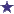# Electrolyte Solution DILUTION/Percentage Strength QuestionDilution / Percentage Strength Question

you have 200ml of a 30% solution. You dilute the solution to 600ml. What is the percent strength of the final solution?

### Comments for Electrolyte Solution DILUTION/Percentage Strength Question

Average RatingRatingPharmacy Dilution/Percent Strength Math Problem by: Anonymous X/200ml = 30/100 (30%) and figure out for X to find the amount of diluent30x200ml = 6000 = 100x X = 60mlSo next, 60/200ml = 30/100 = 30%, so now we can solve for what the percent strength is in a 600ml solution. 60/600 = 10% The percentage strength of a 200ml, 30% solution diluted to 600ml is 10%.